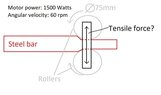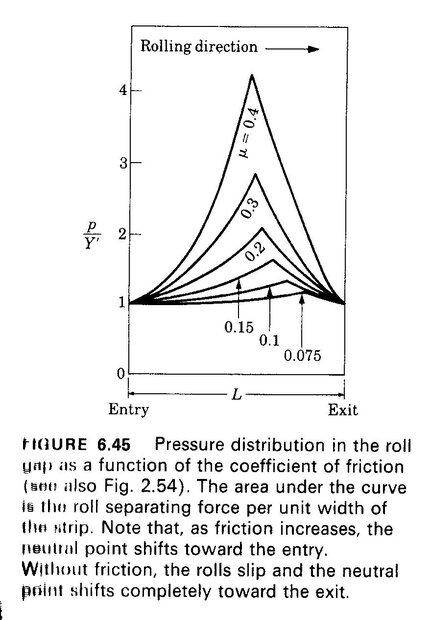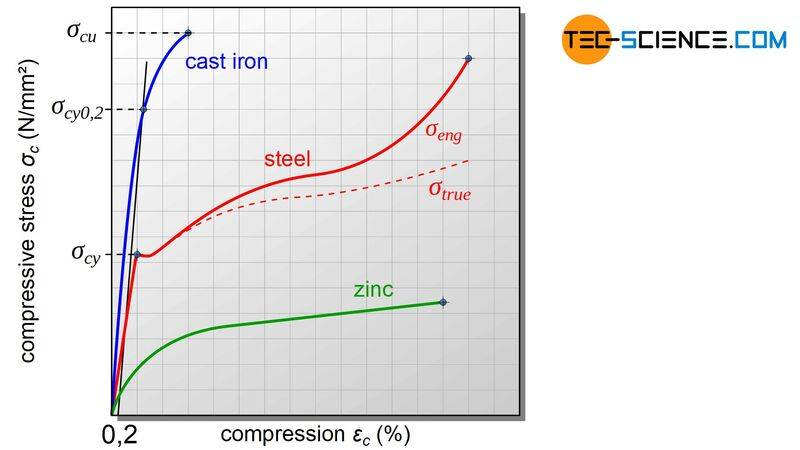# Rolling mill force calculations

Phynight
TL;DR Summary
Trying to figure out the forces involved in squishing a bit of metal between two wheels.
Hi, I'm building a rolling mill for flattening out bits of steel and I'm trying to make sure it's not going to break.
I know that with a 1500w motor geared down to 60rpm (2pi rad/s) i end up with 239Nm of torque.
239Nm working at a radius of 0.0375m gives 6369N of force generated at the edge of the rollers, but that's not the force that would be acting in the vertical plane because where the rollers touch the steel the forces would be mostly horizontal. Or is it? My gut tells me that the forces should be much bigger than that.

Thanks for any help.

#### Attachments

•Screenshot_20221016-220222_WhatsApp.jpg
22.8 KB · Views: 25

Mentor
My copy of Manufacturing Processes for Engineering Materials, by Kalpakjian gives a simplified method of calculating the roll separating force as ##F = Lwp_{av}## where
##L## is the length of the arc of contact
##w## is the width of the roll contact
##p_{av}## is the average contact pressure

The average contact pressure is a function of material strain hardening, amount of thickness reduction, and friction coefficient. For example, the effect of friction coefficient is as follows. Note the large change in roll separating force with friction coefficient.The complete discussion runs about 16 pages in my first edition of this book. It's a little much to summarize for this thread, and the 6th edition of the book is available at a very reasonable price from Amazon: https://www.amazon.com/dp/B09YMFB23Z/?tag=pfamazon01-20. The book is well written, easy to understand, and I highly recommend it. The low cost paperback copy in the Amazon link is for a book printed in India. That could be a problem if you wanted a textbook for class, but should be perfectly acceptable as a reference for a personal project.

•Phynight, berkeman and Lnewqban
Hi, I'm building a rolling mill for flattening out bits of steel and I'm trying to make sure it's not going to break.
You appear to be "coining" the material between two rollers. If you do not need to change the thickness, then the steel can be more economically straightened by the use of three or four rollers. The first three rollers are set to "yield" a long curve in the steel, it will flex back elastically to form a uniform radius curve. The last three rollers are used to reverse the curve, plus a little, so after yielding, it elastically returns to a straight bar, or a flat sheet. The energy needed for straightening by bending, is very much less than by coining, so the equipment can be very much lighter, and lower cost.

There are many examples here.
https://www.ejpmachines.com/machines/straightening_machines.html

Last edited:
•Lnewqban
Gold Member
The vertical force needed will depend on the stress-strain characteristic in compression for the material. For example:If the goal is to flatten the specimen (permanent deformation), you will have to create a compressive stress above the compressive yield strength ##\sigma_{cy}## (on the previous plot, for steel). Anything below that stress value, the deformation will be elastic and the specimen will recover its original shape.

The compressive force ##F## is therefore:
$$F=\sigma A$$
where ##A## is the area of deformation, which I'm not sure how to find for a roller on a plate. It must not be too difficult to find what is usually used in the industry.

If the stress goes above the maximum value (extreme right of the curve), the specimen is destroyed.

If it stays in the elastic portion of the stress-strain curve (initial straight line), the force ##F## will be:
$$F= \left(E\frac{\Delta t}{t_0}\right)A$$
Where ##E## is the Young's modulus, ##t_0## is the initial thickness, and ##\Delta t## is the variation of the thickness. Obviously, the minimum squishing for permanent deformation ##\Delta t_{cy}## will be:
$$\Delta t_{cy} = \frac{\sigma_{cy}}{E} t_0$$

The roller force ##F_x## needed to achieve the compressive will probably be something like:
$$F_x = \frac{F}{C_r}$$
Where ##C_r## is some coefficient of "rolling resistance" at the desired deformation for the desired material. There must be some similar values available from the industry.

•Lnewqban
Phynight
My copy of Manufacturing Processes for Engineering Materials, by Kalpakjian gives a simplified method of calculating the roll separating force as ##F = Lwp_{av}## where
##L## is the length of the arc of contact
##w## is the width of the roll contact
##p_{av}## is the average contact pressure

The average contact pressure is a function of material strain hardening, amount of thickness reduction, and friction coefficient. For example, the effect of friction coefficient is as follows. Note the large change in roll separating force with friction coefficient.
View attachment 315685
The complete discussion runs about 16 pages in my first edition of this book. It's a little much to summarize for this thread, and the 6th edition of the book is available at a very reasonable price from Amazon: https://www.amazon.com/dp/B09YMFB23Z/?tag=pfamazon01-20. The book is well written, easy to understand, and I highly recommend it. The low cost paperback copy in the Amazon link is for a book printed in India. That could be a problem if you wanted a textbook for class, but should be perfectly acceptable as a reference for a personal project.
Looks like I'm going to have to get that book. I was hoping there would be some kind of calculation for the maximum possible output based on motor power but it looks like there going to be a lot more maths to it than that.
Thanks a bunch!

•berkeman